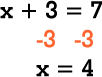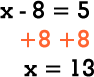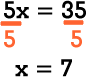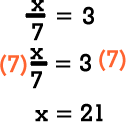To solve a one-step equation, do the inverse of whatever operation is being done to the variable. Because it is an equation, what is done to one side of the equation must be done to the other side of the equation.

 Solve an addition equation by subtraction. Solve a subtraction equation by addition.Solve a multiplication equation by division. Solve a division equation by multiplication.Enter the correct answers for x.

 x = x =
 x = x =

Home